﻿ Using Maple to Study Some Differential Problems

### Using Maple to Study Some Differential Problems

Chii-Huei YuOPEN ACCESSPEER-REVIEWED

## Using Maple to Study Some Differential Problems

Department of Management and Information, Nan Jeon University of Science and Technology, Tainan City, Taiwan

### Abstract

This paper takes the mathematical software Maple as the auxiliary tool to study the differential problems of four types of functions. We can obtain the infinite series forms of any order derivatives of these functions by using binomial theorem and differentiation term by term theorem, and hence greatly reduce the difficulty of calculating their higher order derivative values. On the other hand, we propose two examples to do calculation practically. The research methods adopted in this study involved finding solutions through manual calculations and verifying these solutions by using Maple.

• Yu, Chii-Huei. "Using Maple to Study Some Differential Problems." International Transaction of Electrical and Computer Engineers System 2.2 (2014): 56-60.
• Yu, C. (2014). Using Maple to Study Some Differential Problems. International Transaction of Electrical and Computer Engineers System, 2(2), 56-60.
• Yu, Chii-Huei. "Using Maple to Study Some Differential Problems." International Transaction of Electrical and Computer Engineers System 2, no. 2 (2014): 56-60.

 Import into BibTeX Import into EndNote Import into RefMan Import into RefWorks

### 1. Introduction

The computer algebra system (CAS) has been widely employed in mathematical and scientific studies. The rapid computations and the visually appealing graphical interface of the program render creative research possible. Maple possesses significance among mathematical calculation systems and can be considered a leading tool in the CAS field. The superiority of Maple lies in its simple instructions and ease of use, which enable beginners to learn the operating techniques in a short period. In addition, through the numerical and symbolic computations performed by Maple, the logic of thinking can be converted into a series of instructions. The computation results of Maple can be used to modify our previous thinking directions, thereby forming direct and constructive feedback that can aid in improving understanding of problems and cultivating research interests. Inquiring through an online support system provided by Maple or browsing the Maple website (www.maplesoft.com) can facilitate further understanding of Maple and might provide unexpected insights. As for the instructions and operations of Maple, we can refer to [1-7].

In calculus and engineering mathematics curricula, evaluating the k-th order derivative value f(k)(c) of a function f(x) at x=c , in general, needs to go through two procedures: firstly determining the k-th order derivative f(k)(x) of f(c), and then takinginto f(k)(x). These two procedures will make us face with increasingly complex calculations when calculating higher order derivative values of this function (i.e. k is large), and hence to obtain the answers by manual calculations is not easy. In this paper, we mainly study the differential problems of the following four types of functions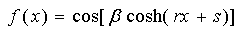(1)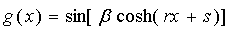(2)(3)(4)

where β,r,s are real numbers. We can determine the infinite series forms of any order derivatives of these functions by using binomial theorem and differentiation term by term theorem; these are the major results of this study (i.e., Theorems 1 and 2), and hence greatly reduce the difficulty of calculating their higher order derivative values. For the study of related differential problems can refer to [8-21]. In addition, we provide two examples to do calculation practically. The research methods adopted in this study involved finding solutions through manual calculations and verifying these solutions by using Maple. This type of research method not only allows the discovery of calculation errors, but also helps modify the original directions of thinking from manual and Maple calculations. Therefore, Maple provides insights and guidance regarding problem-solving methods.

### 2. Main Results

Firstly, we introduce some formulas used in this study.

2.1. Formulas
2.1.1. Taylor Series Expression of the Cosine Function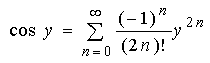, where y is any real number.

2.1.2. Taylor Series Expression of the Sine Function, where y is any real number.

The followings are two important theorems used in this paper.

2.2. Theorems
2.2.1. Binomial Theorem

Suppose x,y are any real numbers, and n is any positive integer. Then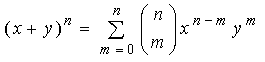, where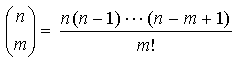for all positive integers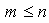, and.

2.2.2. Differentiation Term by Term Theorem ()

For all non-negative integers k, if the functions gk:(a,b)R satisfy the following three conditions：(i) there exists a point x0(a,b) such thatis convergent, (ii) all functions gk(x) are differentiable on open interval (a,b), (iii)is uniformly convergent on (a,b). Then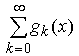is uniformly convergent and differentiable on (a,b). Moreover, its derivative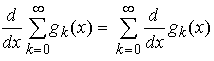.

Before deriving our first major result, we need a lemma.

2.3. Lemma 1

Suppose r,s are real numbers, and n is any positive integer. Then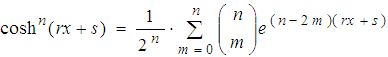(5)

for all xR.

Proof(By binomial theorem)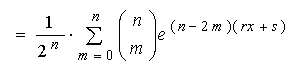The following is the first major result of this article, we obtain the infinite series forms of any order derivatives of the functions (1) and (2).

2.4. Theorem 1

Suppose β,r,s are real numbers, and k is any positive integer.

(1) If the domain of the functionis (−∞,∞). Then the k-th order derivative of f(x),(6)

for all xR.

(2) Suppose the domain of the function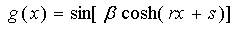is (−∞,∞). The k-th order derivative of g(x),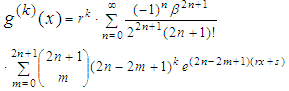(7)

for all xR.

Proof (1) Because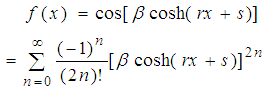(By Formula 2.1.1)(8)

(By Lemma 1)

By differentiation term by term theorem, differentiating k-times with respect to x on both sides of (8), we obtainfor all xR.

(2) Because(By Formula 2.1.2)(9)

(By Lemma 1)

Using differentiation term by term theorem, differentiating k-times with respect to x on both sides of (9), we have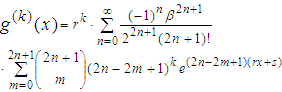for all xR.

To prove the second major result of this study, we also need a lemma.

2.5. Lemma 2

If the assumptions are the same as Lemma 1, then(10)

for all xR.

Proof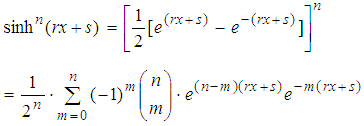(By binomial theorem)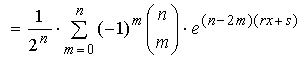2.6. Theorem 2

If the assumptions are the same as Theorem 1.

(1) Let the domain of the functionbe (−∞,∞). Then the -th order derivative of ,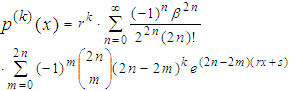(11)

for all xR.

(2) Suppose the domain of the functionis (−∞,∞). Then(12)

for all xR.

Proof (1) Because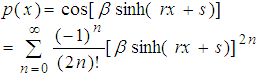(By Formula 2.1.1)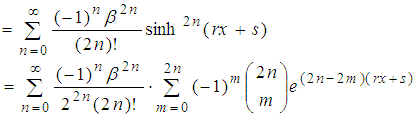(13)

(By Lemma 2)

Also, by differentiation term by term theorem, differentiating k-times with respect to x on both sides of (13), we have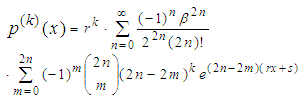for all xR.

(2) Because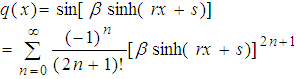(By Formula 2.1.2)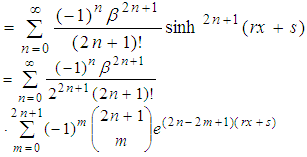(14)

(By Lemma 2)

Using differentiation term by term theorem, differentiating k-times with respect to x on both sides of (14), thenfor all xR.

### 3. Examples

In the following, for the differential problems of the four types of functions in this study, we provide two examples and use Theorems 1,2 to determine the infinite series forms of any order derivatives and some higher order derivative values of these functions. On the other hand, we employ Maple to calculate the approximations of these higher order derivative values and their solutions for verifying our answers.

3.1. Example 1
3.1.1. Suppose the Domain of the Function(15)

is (−∞,∞). Then by (6) of Theorem 1, we obtain any k-th order derivative of f(x),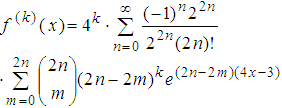(16)

for all xR.

Thus, we can evaluate the 5-th order derivative value of f(x)at x=1/4,(17)

Next, we use Maple to verify the correctness of (17).

>f:=x->cos(2*cosh(4*x-3));

>evalf((D@@5)(f)(1/4),28);

5.51145512574533574051334•105

>evalf(4^5*sum((-1)^n*2^(2*n)/(2^(2*n)*(2*n)!)*sum((2*n)!/(m!*(2*n-m)!)*(2*n-2*m)^5*exp(-4*(n-m)),

m=0.2*n),n=0.infinity),28);

5.51145512574533574051345•105

3.1.2. If the Domain of the Function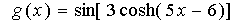(18)

is (−∞,∞). Then by (7) of Theorem 1, we obtain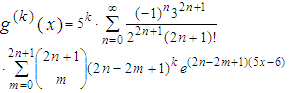(19)

for all xR.

Therefore, the 7-th order derivative value of g(x)at x=1,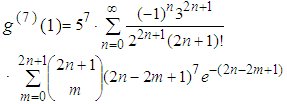(20)

>g:=x->sin(3*cosh(5*x-6));

>evalf((D@@7)(g)(1),24);

3.11674791628708010692687•109

>evalf(5^7*sum((-1)^n*3^(2*n+1)/(2^(2*n+1)*(2*n+1)!)*sum((2*n+1)!/(m!*(2*n-m+1)!)*(2*n-2*m+1)^7*exp(-(2*n-2*m+1)),m=0.(2*n+1)),n=0.infinity),24);

3.11674791628708010692539•109

3.2. Example 2
3.2.1. Assume the Domain of the Function(21)

is (−∞,∞). Using (11) of Theorem 2, we obtain(22)

for all xR.

Thus, the 6-th order derivative value of p(x) at x=1/2,(23)

>p:=x->cos(4*sinh(8*x+6));

>evalf((D@@6)(p)(-1/2),24);

-1.854326252223362289•1012

>evalf(8^6*sum((-1)^n*4^(2*n)/(2^(2*n)*(2*n)!)*sum((-1)^m*(2*n)!/(m!*(2*n-m)!)*(2*n-2*m)^6*exp(4*(n-m)),

m=0.2*n),n=0.infinity),24);

1.854326252223362269•1012

3.2.2. If the Domain of the Function(24)

is (−∞,∞). Using (12) of Theorem 2, we obtain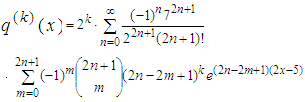(25)

for all xR.

Hence, we can determine the 6-th order derivative value of q(x) at x=3,(26)

>q:=x->sin(7*sinh(2*x-5));

>evalf((D@@6)(q)(3),20);

-9.468885110895329•107

>evalf(2^6*sum((-1)^n*7^(2*n+1)/(2^(2*n+1)*(2*n+1)!)*sum((-1)^m*(2*n+1)!/(m!*(2*n-m+1)!)*(2*n-2*m+1)^6*exp(2*n-2*m+1),m=0.(2*n+1)),n=0.infinity),20);

-9.468885110895331•107

### 4. Conclusion

In this article, we provide a new technique to determine any order derivatives of some types of functions. We hope this technique can be applied to solve another differential problems. On the other hand, the binomial theorem and the differentiation term by term theorem play significant roles in the theoretical inferences of this study. In fact, the applications of these two theorems are extensive, and can be used to easily solve many difficult problems; we endeavor to conduct further studies on related applications. In addition, Maple also plays a vital assistive role in problem-solving. In the future, we will extend the research topic to other calculus and engineering mathematics problems and solve these problems by using Maple. These results will be used as teaching materials for Maple on education and research to enhance the connotations of calculus and engineering mathematics.

### References

  R. J. Stroeker and J. F. Kaashoek, Discovering mathematics with Maple: an interactive exploration for mathematicians, engineers and econometricians, Birkhauser Verlag, Basel, 1999.In article CrossRef  M. L. Abell and J. P. Braselton, Maple by example, 3rd ed., Elsevier Academic Press, New York, 2005.In article  C. Tocci and S. G. Adams, Applied Maple for engineers and scientists, Artech House, Boston, 1996.In article  C. T. J. Dodson and E. A. Gonzalez, Experiments in mathematics using Maple, Springer-Verlag, New York, 1995.In article CrossRef  D. Richards, Advanced mathematical methods with Maple, Cambridge University Press, New York, 2002.In article  F. Garvan, The Maple book, Chapman & Hall/CRC, London, 2001.In article CrossRef  J. S. Robertson, Engineering mathematics with Maple, McGraw-Hill, New York, 1996.In article  M. A. Patterson, M. Weinstein, and A. V. Rao, “An efficient overloaded method for computing derivatives of mathematical functions in MATLAB,” ACM Transactions on Mathematical Software, vol. 39, issue. 3, 2013.In article  M. Wagner, A. Walther, and B. J. Schaefer, “On the efficient computation of high-order derivatives for implicitly defined functions,” Computer Physics Communications, vol. 181, issue. 4, pp. 756–764, 2010.In article CrossRef  A. Griewank and A. Walther, Evaluating derivatives: principles and techniques of algorithmic differentiation, 2nd ed., SIAM, Philadelphia, 2008.In article CrossRef  C. -H. Yu, “A study on the differential problem,” International Journal of Research in Aeronautical and Mechanical Engineering, vol. 1, issue. 3, pp. 52-57, 2013.In article  C. -H. Yu, “The differential problem of two types of functions,” International Journal of Computer Science and Mobile Computing, vol. 2, issue. 7, pp. 137-145, 2013.In article  C. -H. Yu, “Evaluating the derivatives of two types of functions,” International Journal of Computer Science and Mobile Applications, vol. 1, issue. 2, pp. 1-8, 2013.In article  C. -H. Yu, “Evaluating the derivatives of trigonometric functions with Maple,” International Journal of Research in Computer Applications and Robotics, vol. 1, issue. 4, pp. 23-28, 2013.In article  C.-H. Yu, “Application of Maple on solving the differential problem of rational functions,” Applied Mechanics and Materials, vols. 479-480, pp 855-860, 2013.In article CrossRef  C. -H. Yu, “Application of Maple on the differential problem,” Universal Journal of Applied Science, vol. 2, no. 1, pp. 11-18, 2014.In article  C. -H. Yu, “Studying the differential problem with Maple,” Computer Science and Information Technology, vol.1, no.3, pp. 190-195, 2013.In article  C. -H. Yu, “ A study on some differential problems with Maple,” Proceedings of 6th IEEE/International Conference on Advanced Infocomm Technology, Taiwan, no. 00291, July 2013.In article  C. -H. Yu, “Application of Maple on solving some differential problems,” Proceedings of IIE Asian Conference 2013, Taiwan, vol. 1, pp. 585-592, July 2013.In article  C. -H. Yu, “The differential problem of four types of functions,” Academic Journal of Kang-Ning, vol. 15, pp. 51-63, 2013.In article  C. -H. Yu, “A study on the differential problem of some trigonometric functions,” Journal of Jen-Teh, vol. 10, pp. 27-34, 2013.In article  T. M. Apostol, Mathematical analysis, 2nd ed., Addison-Wesley Publishing, Boston, p230, 1975.In article